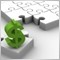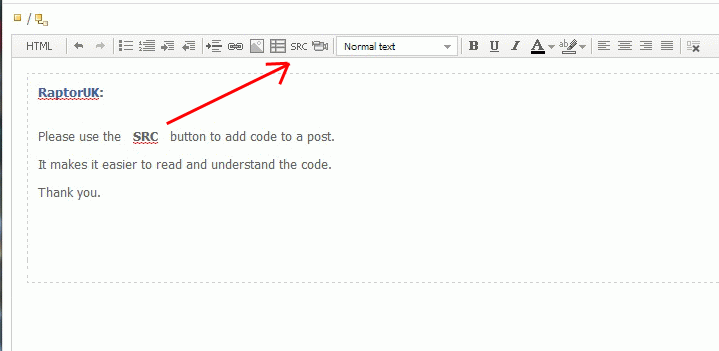# pls help !!! my ea open only sell order36

i tried to test my ea by the strategy tester , but it open only sell order.

what 's wrong?

``` //+------------------------------------------------------------------+

//|                                                 200SMA_CCI25.mq4 |

//|                        Copyright 2015, MetaQuotes Software Corp. |

//|                                             https://www.mql5.com |

//+------------------------------------------------------------------+

#property version   "1.00"

#property strict

extern int    MGNo    = 555;

extern double risk=0.2;

extern   int    STEP=1;

extern   int    ConRisk=100;

extern int   TP   = 500;

bool   Trailing       = true;

extern double TrailingStop    = 100;

bool DisplayStatus=false;

bool result;

double p,o,s,tp,markh,markl,marktp,target1,target2,mark,marksl,nsl,ntp;

int once=0,tarpip,onlyonce=0,cnt,order,ch;

string ord;

int start()

{

static datetime tmp;

double stl=MarketInfo(Symbol(),MODE_STOPLEVEL);

tarpip=ConRisk;

//if(ConRisk>0)tarpip=ConRisk;

//if(MinRisk>0)tarpip=MinRisk;

double maxl=MarketInfo(Symbol(),MODE_MAXLOT);

double minl=MarketInfo(Symbol(),MODE_MINLOT);

double tv=MarketInfo(Symbol(), MODE_TICKVALUE);

double MoneyUse=risk*MathFloor(AccountBalance()/STEP)*STEP;

//risk=0.2*AccountBalance();

double x=MoneyUse/tarpip;

double lot=x/tv;

if(lot>maxl)lot=maxl;

if(lot<minl)lot=minl;

double SMA200=iMA(Symbol(),0,200,0,MODE_SMA,PRICE_CLOSE,1);

double c1=Close;

double cci1=iCCI(Symbol(),0,25,PRICE_TYPICAL,1);

double cci2=iCCI(Symbol(),0,25,PRICE_TYPICAL,2);

if(c1>SMA200 && cci1>-100 && cci2<=-100)

{

order=1;

}

else {order=0;ord="";}

if(c1<SMA200 && cci1<100 && cci2>=100)

{

order=-1;

ord="sell";

}

else {order=0;ord="";}

if (tmp < Time )

{

if(OrdersTotal()==0)

{

if(order==1)

{

if(ch>0){order=0;}

}

if(order==-1)

{

ch=OrderSend(Symbol(),OP_SELL,lot,Bid,0,Bid+ConRisk*Point,Bid-TP*Point,"",555,0,clrNONE);

if(ch>0){order=0;}

}

}

if(Trailing)

{

for(cnt=0;cnt<OrdersTotal();cnt++)

{

continue;

if(OrderType()<=OP_SELL &&   // check for opened position

OrderSymbol()==Symbol()&& OrderMagicNumber()==MGNo)  // check for symbol

{

//--- long position is opened

{

//--- check for trailing stop

if(TrailingStop>0)

{

if(Bid-OrderOpenPrice()>Point*TrailingStop)

{

if(OrderStopLoss()<Bid-Point*TrailingStop)

{

//--- modify order and exit

if(!OrderModify(OrderTicket(),OrderOpenPrice(),Bid-Point*TrailingStop,OrderTakeProfit(),0,Green))

Print("OrderModify error ",GetLastError());

return(0);

}

}

}

}

else // go to short position

{

//--- check for trailing stop

if(TrailingStop>0)

{

{

{

//--- modify order and exit

Print("OrderModify error ",GetLastError());

return(0);

}

}

}

}

}

}

}

tmp = Time ;

}

Comment(c1,"/",SMA200,"/",cci1,"/",cci2);

return(0);

}```10148

Please use the SRC button when posting code. I've fixed it for you this time36

sorry

and

thank you10148

oilyjaah:

sorry

and

thank you

No problem :)

Are you seeing any errors in your journal?36

no

and realy would like to

what's wrong?

pls help10148

oilyjaah:

no

and realy would like to

what's wrong?

pls help

Your problem is the way you've coded the conditional logic for the buy sell. Currently, if the buy condition is met, it then drops down to check the sell condition. As this is false, it moves to the "else" of the sell condition which resets the order var to zero, hence no trade is opened. Try something like this instead:

```   if(c1>SMA200 && cci1>-100 && cci2<=-100)
{
order=1;
}
else if(c1<SMA200 && cci1<100 && cci2>=100)
{
order=-1;
ord="sell";
}
else
{
order=0;
ord="";
}
```

You could also streamline your OrderSend() code so you don't need to check for buy/sell again but it should work OK as it is, just not as efficient.

Cheers
Stu36

thank you

i will try10148

oilyjaah:

thank you

i will try

Very welcome :)14

```void TechnicalAnalysis3x18()

{

if ((iStochastic(NULL, PERIOD_M15,5,3,3,MODE_SMA,1,MODE_MAIN,current+1)<20

&& (iStochastic(NULL, PERIOD_M15,5,3,3,MODE_SMA,1,MODE_MAIN,current+1)<20 > 20)

&& (iStochastic(NULL, PERIOD_M15,5,3,3,MODE_SMA,1,MODE_MAIN,current+1) > (iStochastic(NULL, PERIOD_M15,5,3,3,MODE_SMA,1,MODE_SIGNAL,current+1)))

&& (iStochastic(NULL, PERIOD_M15,5,3,3,MODE_SMA,1,MODE_SIGNAL,current)<50)))

{

IfOrderDoesNotExist21();

}

}

void IfOrderDoesNotExist21()

{

bool exists = false;

for (int i=OrdersTotal()-1; i >= 0; i--)

{

if (OrderType() == OrderType21 && OrderSymbol() == Symbol() && OrderMagicNumber() == 1)

{

exists = true;

}

}

else

{

Print("OrderSelect() error - ", ErrorDescription(GetLastError()));

}

if (exists == false)

{

}

}

{

if (BuyStoploss12 == 0) SL = 0;

if (BuyTakeprofit12 == 0) TP = 0;

int ticket = -1;

if (true)

else

if (ticket > -1)

{

if (true)

{

bool sel = OrderSelect(ticket, SELECT_BY_TICKET);

bool ret = OrderModify(OrderTicket(), OrderOpenPrice(), SL, TP, 0, Blue);

if (ret == false)

Print("OrderModify() error - ", ErrorDescription(GetLastError()));

}

LimitOpenOrders11();

}

else

{

Print("OrderSend() error - ", ErrorDescription(GetLastError()));

}

}```14

Thank youModerator
24775

MrLets:

Please use the code button when inserting code. I have edited it for you this time.

Also please avoid using huge gaps between lines, it can make the code difficult to read.